# Working with Gridded Data II ¶

In :
import numpy as np
import xarray as xr
import holoviews as hv
import geoviews as gv
import geoviews.feature as gf
from cartopy import crs as ccrs

hv.notebook_extension()
%output size=200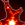The main strength of HoloViews and its extensions (like GeoViews) is the ability to quickly explore complex datasets by declaring lower-dimensional views into a higher-dimensional space. In HoloViews we refer to the interface that allows you to do this as the conversion API. To begin with we will load a multi-dimensional dataset of surface temperatures for different "realizations" (modelling parameters) using XArray :

In :
xr_ensembles = xr.open_dataset('./sample-data/ensembles.nc')
xr_ensembles

Out:
<xarray.Dataset>
Dimensions:                  (bnds: 2, latitude: 145, longitude: 192, realization: 13, time: 6)
Coordinates:
* realization              (realization) int32 0 1 2 3 4 5 7 8 9 10 11 12 13
* time                     (time) datetime64[ns] 2011-08-16T12:00:00 ...
* latitude                 (latitude) float32 -90.0 -88.75 -87.5 -86.25 ...
* longitude                (longitude) float32 0.0 1.875 3.75 5.625 7.5 ...
forecast_reference_time  (realization, time) datetime64[ns] 2011-07-18 ...
Dimensions without coordinates: bnds
Data variables:
surface_temperature      (realization, time, latitude, longitude) float64 210.1 ...
latitude_longitude       int32 -2147483647
time_bnds                (time, bnds) float64 3.645e+05 3.652e+05 ...
Attributes:
source: Data from Met Office Unified Model
um_version: 7.6
Conventions: CF-1.5

As we saw in the Gridded Datasets I Tutorial we can easily wrap this xarray data structure as a HoloViews Dataset:

In :
kdims = ['realization', 'longitude', 'latitude', 'time']
vdims = ['surface_temperature']
dataset = gv.Dataset(xr_ensembles, kdims=kdims, vdims=vdims, crs=ccrs.PlateCarree())
dataset

Out:
:Dataset   [realization,longitude,latitude,time]   (surface_temperature)

From the repr we can immediately see the list of key dimensions (time, realization, longitude and latitude) and the value dimension of the cube (surface_temperature). However, unlike most other HoloViews Elements, the  Dataset  Element does not display itself visually. This is because it can be n-dimensional and therefore does not have any specific straightforward visual representation on a 2D display. To view the cube, we first have to transform it into individually visualizable chunks. Before doing so, we will want to supply a custom value formatter for the time dimension so that it is readable by humans:

In :
hv.Dimension.type_formatters[np.datetime64] = '%Y-%m-%d'


# Conversions ¶

A HoloViews Dataset is a wrapper around a complex multi-dimensional datastructure which allows the user to convert their data into individually visualizable views, each usually of lower dimensionality. This is done by grouping the data by some dimension and then casting it to a specific Element type, which visualizes itself.

The  dataset.to  interface makes this especially easy. To use it, you supply the Element type that you want to view the data as and the key dimensions of that view and it will figure out the rest. Depending on the type of Element, you can specify one or more dimensions to be displayed. GeoViews provides a set of GeoElements that allow you to display geographic data on a cartographic projection, but you can use any Elements from HoloViews for non-geographic plots.

Recall that the cube we are working with has 4 coordinate dimensions (or key dimensions as they are known in HoloViews) -- time, realization, longitude, and latitude. For our purposes, a geographic plot is defined as a plot that has longitude along the x axis and latitude along the y axis. To declare a two-dimensional geographic plot, we therefore simply request a  gv.Image  plot with  longitude  and  latitude  as key dimensions. There is one value dimension (vdim) available,  surface_temperature  , and any remaining key dimensions (  time  and  realization  in this case) are assigned to a  HoloMap  data structure by default. The resulting  HoloMap  gives you widgets automatically to allow you to explore the data across the two "remaining" key dimensions (those not mapped onto axes of the image):

In :
geo_dims = ['longitude', 'latitude']
(dataset.to(gv.Image, geo_dims) * gf.coastline)[::5, ::5]

Out:

In this way we can visualize the geographic data in a number of ways, currently either as an  Image  (as above) or as  LineContours  ,  FilledContours  , or  Points  :

In :
%%opts Points [color_index=2 size_index=None] (cmap='jet')
hv.Layout([dataset.to(el, geo_dims)[::10, ::10] * gf.coastline
for el in [gv.FilledContours, gv.LineContours, gv.Points]]).cols(1)

Out:

Note that by default the conversion interface will automatically expand all the individual Elements, which can take some time if the data is very large. Instead we can also request the objects to be expanded dynamically using the  dynamic  keyword:

In :
dataset.to(gv.Image, geo_dims, dynamic=True) * gf.coastline

Out:

Using  dynamic  mode means that the data for each frame is only extracted when you're actually viewing that part of the data, which can have huge benefits in terms of speed and memory consumption. However, it relies on having a running Python process to render and serve each image, and so it cannot be used when generating static HTML output such as for the GeoViews web site.

## Non-geographic views ¶

So far we have focused entirely on geographic views of the data, plotting the data on a projection. However the conversion interface is completely general, allowing us to slice and dice the data in any way we like. The simplest example of this capability is simply a view showing the temperature over time for each realization, longitude, and latitude coordinate:

In :
%%opts Curve [xrotation=25] NdOverlay [fig_size=200 aspect=1.2]
dataset.to(hv.Curve, 'time', dynamic=True).overlay('realization')

Out:

Note that the longitude slider will have no effect, if latitude is -90 or +90, since there is only one data point for the North or South poles (regardless of the declared longitude). Here the  .overlay  gives a different curve for each  realization  ; without it all  realization  values would be pooled together.

We can also make non-geographic 2D plots, for instance as a  HeatMap  over time and realization, at a specified longitude and latitude:

In :
%%opts HeatMap [show_values=False colorbar=True]
dataset.to(hv.HeatMap, ['realization', 'time'], dynamic=True)

Out:

## Lower-dimensional views ¶

So far all the conversions shown have incorporated each of the available coordinate dimensions explicitly. However, often times we want to see the spread of values along one or more dimensions, pooling all the other dimensions together. A simple example of this is a box plot where we might want to see the spread of surface_temperature on each day, pooled across all latitude and longitude coordinates. To pool across particular dimensions, we can explicitly declare the "map" dimensions, which are the key dimensions of the HoloMap container rather than those of the Elements contained in the HoloMap. By explicitly declaring no dimensions to  groupby  , we can tell the conversion interface to pool across all dimensions except the particular key dimension(s) supplied, in this case the  'time'  (plot A ) and  'realization'  (plot B ):

In :
%%opts BoxWhisker [xrotation=25 bgcolor='w']
hv.Layout([dataset.to.box(d, None, []) for d in ['time', 'realization']])

Out: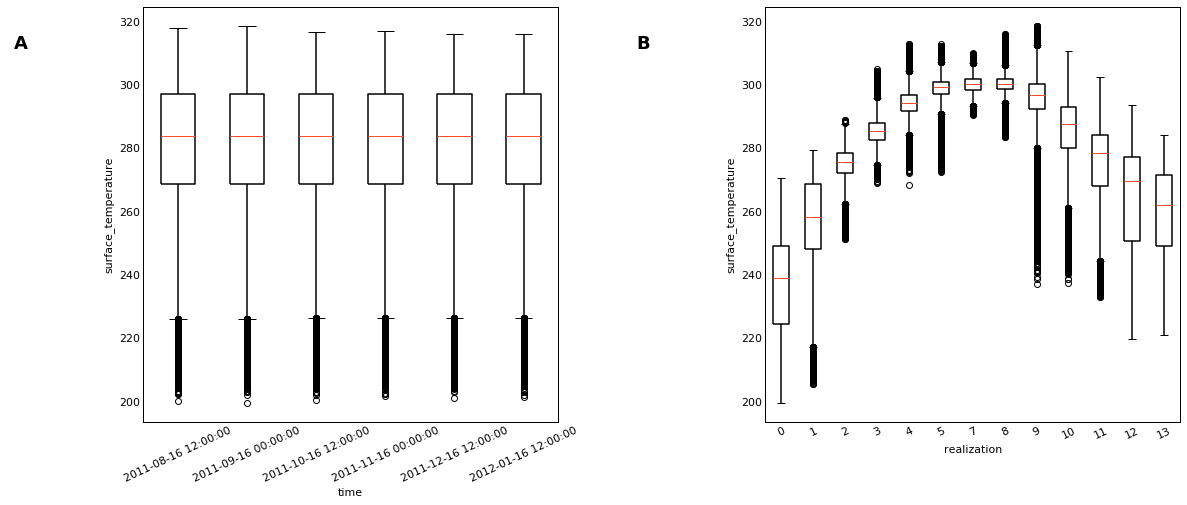This approach also gives us access to other statistical plot types. For instance, with the  seaborn  library installed, we can use the  Distribution  Element, which visualizes the data as a kernel density estimate. In this way we can visualize how the distribution of surface temperature values varies over time and the model realizations. We do this by omitting 'latitude' and 'longitude' from the list of dimensions, generating a lower-dimensional view into the data, where a temperature histogram is shown for every  'realization'  and  'time'  , using  GridSpace  :

In :
%opts GridSpace [shared_xaxis=True]
%opts Distribution [bgcolor='w' show_grid=False xticks=[220, 300]]
try:
import seaborn
grid = dataset.to.distribution(groupby=['realization', 'time']).grid()
except:
grid = None
grid

Out: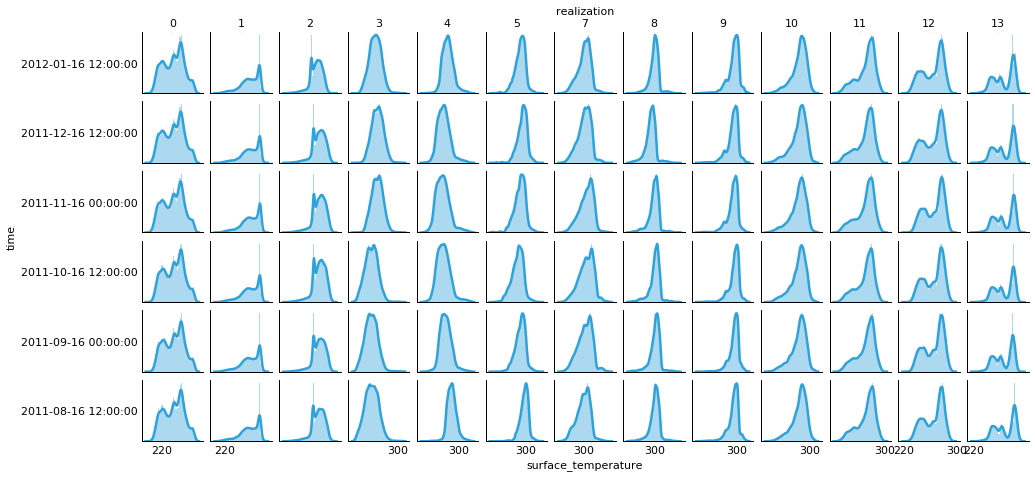## Reducing the data ¶

So far all the examples we have seen have displayed all the data in some way or another. Another way to explore a dataset is to explicitly reduce the dimensionality or select subregions of a dataset. There are two main ways to do this---either we explicitly select a subset of the data, or we collapse a dimension using an aggregation function, e.g. by computing a mean along a particular dimension.

### Selecting slices ¶

Using the  select  method we can easily select ranges of coordinates in the dataset. Unfortunately, the select method does not currently know that latitude and longitude are cyclic, so instead we have to select regions at both ends of the prime meridian (0$^\circ$ longitude) and overlay them. In this way we can stitch together multiple cubes or xarrays or simply view specific subregions:

In :
northern = dataset.select(latitude=(25, 75))
(northern.select(longitude=(260, 305)).to(gv.Image, geo_dims) *
northern.select(longitude=(330, 362)).to(gv.Image, geo_dims) *
gf.coastline)[::5, ::5]

Out:

### Selecting a particular coordinate ¶

To examine one particular coordinate, we can  select  it, cast the data to Curves, reindex the data to drop the now-constant latitude and longitude dimensions, and overlay the remaining 'realization' dimension:

In :
%opts NdOverlay [xrotation=25 aspect=2  legend_position='right' legend_cols=2] Curve (color=Palette('Set1'))
dataset.select(latitude=0, longitude=0).to(hv.Curve, ['time']).reindex().overlay()

Out: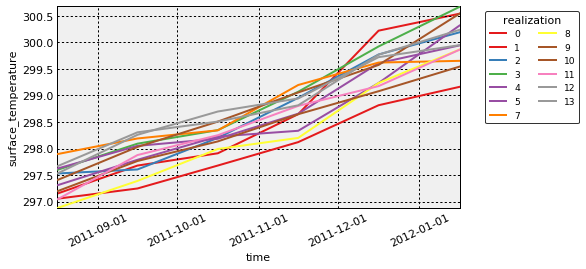### Aggregating coordinates ¶

Another option is to aggregate over certain dimensions, so that we can get an idea of distributions of temperatures across all latitudes and longitudes. Here we compute the mean temperature and standard deviation by latitude and longitude, casting the resulting collapsed view to a Spread Element:

In :
%output size=100
hv.Spread(dataset.aggregate('latitude', np.mean, np.std)) +\
hv.Spread(dataset.aggregate('longitude', np.mean, np.std))

Out: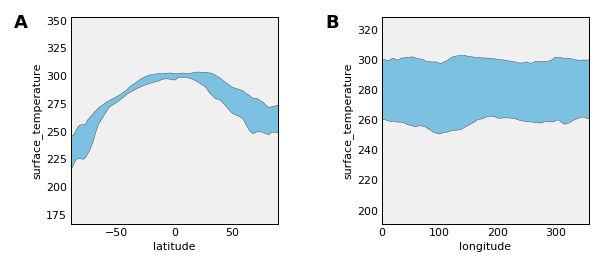As you can see, with GeoViews and HoloViews it is very simple to select precisely which aspects of complex, multidimensional datasets that you want to focus on.